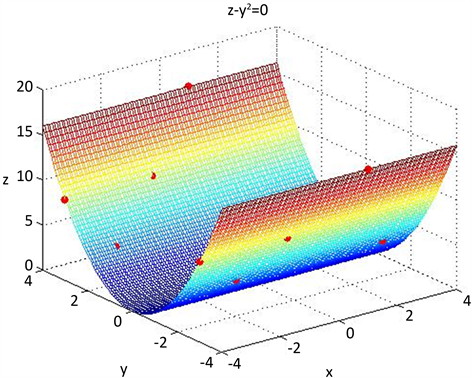# 定义于抛物柱面上的多元Lagrange插值问题Multivariate Lagrange Interpolation Defined on Parabolic Cylinder

• 全文下载: PDF(1653KB)    PP.1039-1044   DOI: 10.12677/AAM.2017.69125
• 下载量: 573  浏览量: 807

Based on the results of two-element function Lagrange interpolation, the only solvable problem of ternary function Lagrange interpolation node group is studied. This paper presents the basic con-cept of the Lagrange interpolation unique solvable node group, which is defined on the parabolic cylinder surface, and studies some basic theory and topological structure of the Lagrange interpola-tion unique solvable node group defined on the parabolic cylinder. The method of adding conic sur-face for constructing the Lagrange interpolating solvable node group which is defined on the para-bolic cylindrical surface is obtained. All of these methods are constructed by means of superposition, so it is very convenient for compiling computer algorithm program and then automatically com-pleting interpolation in the computer to solve the structure of node group and get interpolation format. Finally, an example is given to illustrate the effectiveness of the proposed algorithm.

1. 引言

2. 基本定义和基本定理

$m$ 为如上所定义， $r=\left(\begin{array}{c}n+5\\ 3\end{array}\right)$ ，结点组 $Α={\left\{{Q}_{i}\right\}}_{i=1}^{m}\not\subset F$ 关于 ${P}_{n}^{\left(3\right)}$ 的一个唯一可解结点组，而 $Β={\left\{{Q}_{i}\right\}}_{i=m+1}^{r}$ 是定义于 $F$ 的一个 $n+2$ 次唯一可解结点组，则 ${\left\{{Q}_{i}\right\}}_{i=1}^{r}=Α\cup Β$ 必定构成 ${P}_{n+2}^{\left(3\right)}$ 的唯一可解结点组。

$Α={\left\{{Q}_{i}\right\}}_{i=1}^{{\left(n+1\right)}^{2}}$$F$ 上的 $n$ 次插值唯一可解结点组，平面 $p\left(x,y,z\right)\cap \text{A}=\varphi$$F$ 横截相交于圆锥曲线 $C\left(x,y,z\right)$$\text{B}$ 是定义于圆锥曲线 $C\left(x,y,z\right)$ 上的一个 $n+1$ 次唯一可解结点组，则 $\text{A}\cup \text{B}$ 必定构成定义于 $F$ 上的一个 $n+1$ 次唯一可解结点组。

3. 定理的证明

${p}_{1}\left({Q}_{i}\right)={f}_{i},i=1,\cdots ,m$

${p}_{2}\left({Q}_{i}\right)=\frac{{f}_{i}-{p}_{1}\left({Q}_{i}\right)}{{y}_{i}{}^{2}-2p{z}_{i}}\left(p>0\right),\text{\hspace{0.17em}}i=m+1,\cdots ,r$

$\stackrel{˜}{p}\left(x,y,z\right)={p}_{1}\left(x,y,z\right)+\left({y}^{2}-2pz\right){p}_{2}\left(x,y,z\right)\text{\hspace{0.17em}}\left(p>0\right)$ (2.1)

$\stackrel{˜}{p}\left(x,y,z\right)=\left({y}^{2}-2px\right){p}_{2}\left(x,y,z\right)\in {p}_{n}^{\left(3\right)}\left(p>0\right)$

$p\left(x,y,z\right)=\stackrel{˜}{p}\left(x,y,z\right)=\left({y}^{2}-2pz\right){p}_{2}\left(x,y,z\right)\in {p}_{n}^{\left(3\right)}\left(p>0\right)$

$p\left(x,y,z\right)$ 在抛物柱面 $F$ 上恒取值为零。

4. 实验算例

$p\left(x,y,z\right)={a}_{1}{x}^{2}+{a}_{2}{y}^{2}+{a}_{3}{z}^{2}+{a}_{4}xy+{a}_{5}xz+{a}_{6}yz+{a}_{7}x+{a}_{8}y+{a}_{9}z+{a}_{10}$

$A=\left[\begin{array}{cccccccccc}0& 0& 1& 0& 0& 0& 0& 0& 1& 1\\ 9& 4& 16& -6& 12& -8& 3& -2& 4& 1\\ 9& 4& 16& -6& -12& 8& -3& 2& 4& 1\\ 4& 4& 16& 4& -8& -8& -2& -2& 4& 1\\ 1& 9& 81& 3& -9& -27& -1& -3& 9& 1\\ 1& 9& 81& -3& -9& 27& -1& 3& 9& 1\\ 1& 16& 256& 4& 16& 64& 1& 4& 16& 1\\ 1& 16& 256& -4& 16& -64& 1& -4& 16& 1\\ 16& 9& 81& -12& -36& 27& -4& 3& 9& 1\\ 16& 9& 81& 12& -36& -27& -4& -3& 9& 1\end{array}\right]$ , $X=\left[\begin{array}{c}{a}_{1}\\ {a}_{2}\\ {a}_{3}\\ a{}_{4}\\ {a}_{5}\\ {a}_{6}\\ {a}_{7}\\ {a}_{8}\\ {a}_{9}\\ {a}_{10}\end{array}\right]$ , $B=\left[\begin{array}{c}1\\ \sqrt{29}\\ \sqrt{29}\\ 2\sqrt{6}\\ \sqrt{91}\\ \sqrt{91}\\ \sqrt{273}\\ \sqrt{273}\\ \sqrt{106}\\ \sqrt{106}\end{array}\right]$Figure 1. Parabolic cylinder point taking

$\begin{array}{c}f\left(x,y,z\right)=0.0972{x}^{2}+0.1116{y}^{2}-0.0124{z}^{2}+0.0468xz\\ \text{\hspace{0.17em}}\text{\hspace{0.17em}}-0.1873x+1.0834z-0.0710\end{array}$

  De Boor, C. and Ron, A. (1990) On Multivariate Polynomial Interpolation. Constructive Approximation, 6, 287-302. https://doi.org/10.1007/BF01890412  梁学章. 关于多元函数的插值与逼近[J]. 高等学校计算数学学报, 1979(1): 123-124.  梁学章. 二元插值的适定结点组与迭加插值法[J]. 吉林大学自然科学学报, 1979(1): 27-32.  梁学章, 张洁琳, 崔利宏. 多元Lagrange插值与Cayley-Bacharach定理[J]. 高等数学计算数学学报, 2005(27): 276-281.  梁学章, 张明, 张慧杰, 等. 关于球面上的Lagrange插值[J]. 高等学校计算数学学报, 2011(33): 169-177.  Liang, X.Z., Wang, R.H., Cui, L.H., et al. (2006) Some Researches on Trivariate Lagrange Interpolation. Journal of Computational and Applied Mathematics, 195, 192-205. https://doi.org/10.1016/j.cam.2005.03.083  梁学章, 李强. 多元逼近[M]. 北京: 国防工业出版社, 2005.  Castell, W.Z., Fernandez, N.L. and Xu, Y. (2007) Polynomial Interpolation on the Unit Sphere II. Advances in Computational Mathematics, 26, 155-171 https://doi.org/10.1007/s10444-005-7510-5